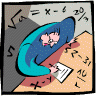## Mathematics, Department of## Dissertations, Theses, and Student Research Papers in Mathematics

8-2015

#### Comments

A DISSERTATION Presented to the Faculty of The Graduate College at the University of Nebraska In Partial Fulfillment of Requirements For the Degree of Doctor of Philosophy, Major: Mathematics, Under the Supervision of Professor Stephen Hartke. Lincoln, Nebraska: August, 2015

Copyright (c) 2015 Sarah Lynne Behrens

#### Abstract

The center of a graph is the set of vertices whose distance to other vertices is minimal. The centralizing number of a graph G is the minimum number of additional vertices in any graph H where V(G) is the center of H. Buckley, Miller, and Slater and He and Liu provided infinite families of graphs with each centralizing number. We show the number of graphs with each nonzero centralizing number grows super-exponentially with the number of vertices. We also provide a method of altering graphs without changing the centralizing number and give results about the centralizing number of dense graphs.

The degree sequence of a (hyper)graph is the list of the number of edges containing each vertex. A t-switch replaces t edges with t new edges while maintaining the same degree sequence. For graphs, it has been repeatedly shown that any realization of a degree sequence can be turned into any other realization by a sequence of 2-switches. However, Gabelman provided an example to show 2-switches are not sufficient for k-graphs with k ≥ 3. We classify all pairs of 3-graphs that do not admit a 2-switch but differ by a 3-switch. We use this to provide support that 2-switches and a 3-switch are sufficient for 3-graphs.

Given graphs G and H, G is H-saturated if G does not contain H as a subgraph, but H is a subgraph of G+e for any e not in E(G). While this is well defined for subgraphs, the similar definition is not well defined for induced subgraphs. To avoid this, Martin and Smith defined the induced-saturation number using trigraphs. We show that the induced-saturation number of stars is zero. This implies the existence of graphs that are star induced-saturated. We introduce the parameter indsat*(n,H) which is the minimum number of edges in an H-induced-saturated graph, when one exists. We provide bounds for indsat*(n,K1,3) and compute it exactly for infinitely many n.

Adviser: Stephen Hartke

COinS